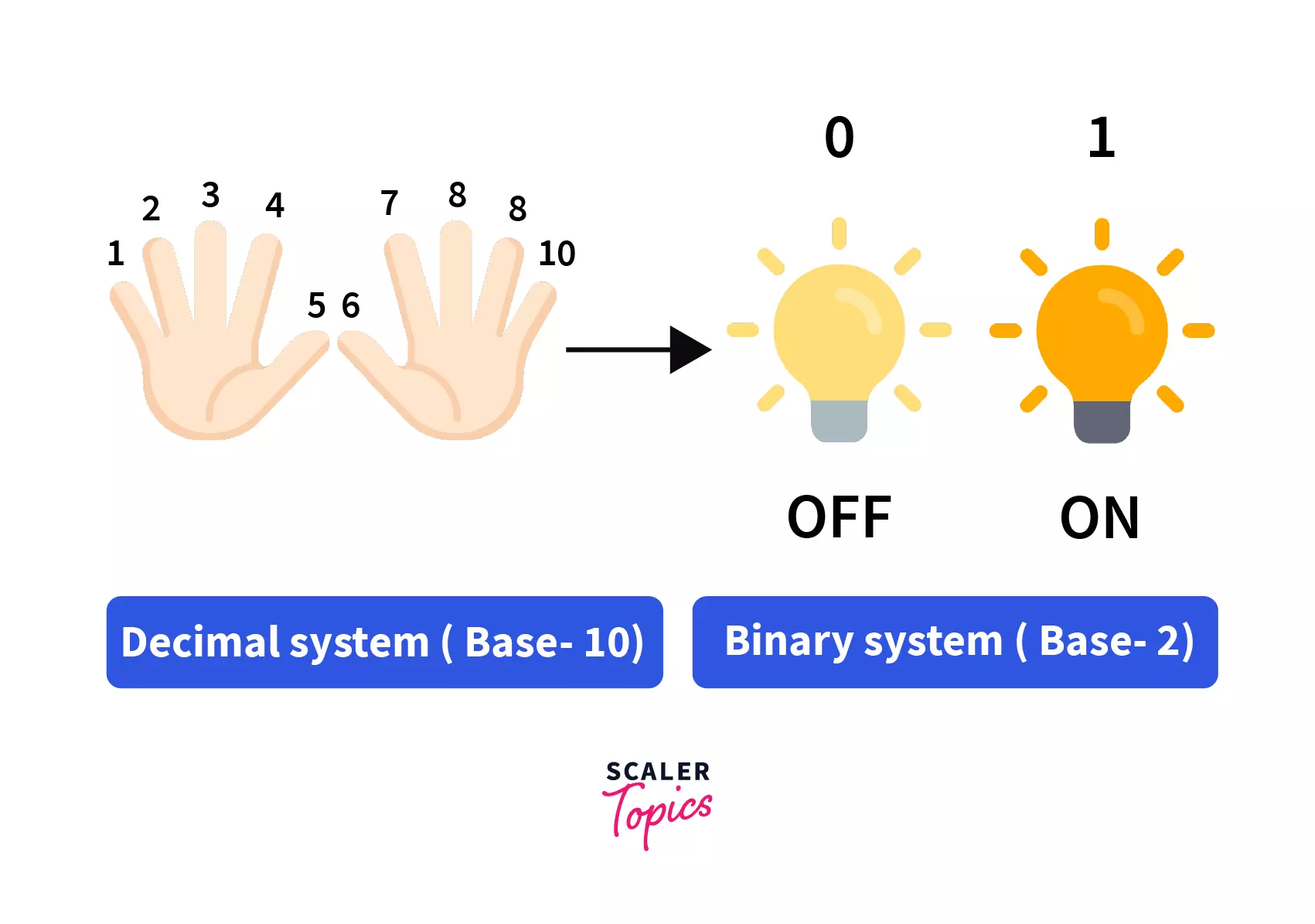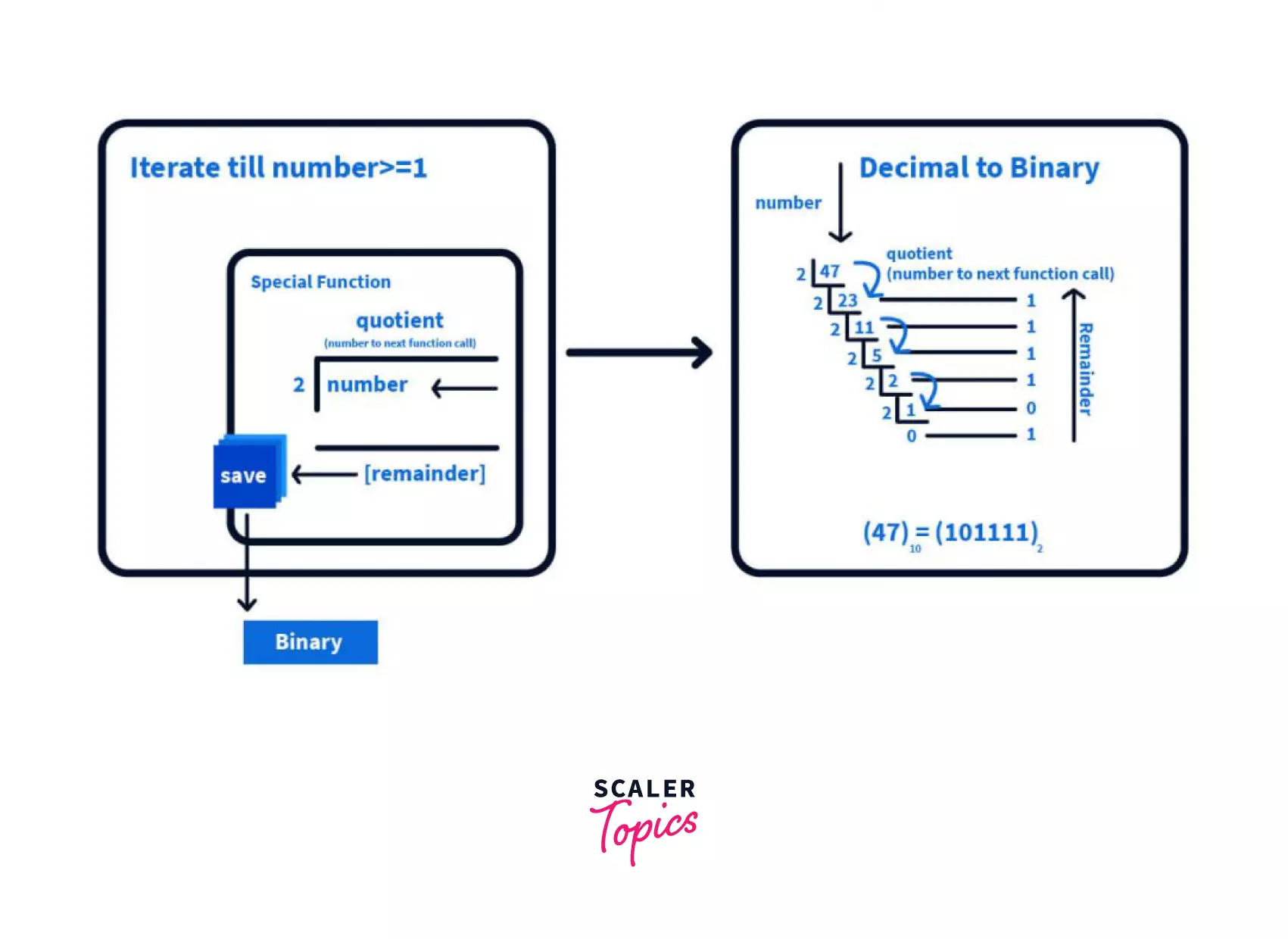Convert Decimal to Binary in Python

Learn how to convert decimal to binary in Python.

Introduction

Binary is one of the most important foundational aspects of Computers and other Digital Systems. As we humans use languages to understand and communicate with each other, Computers and other Digital Systems use Binary. It is a base-2 number system having only two numbers, 0 & 1 which corresponds to ON & OFF states that your computer can understand.

As normal humans have 10 fingers to represent a simple number system called Decimal, computers have these ON & OFF states representing Binary. So to understand and interpret the binary we need some technique to convert binary code into decimal (human-readable) code and vice versa. Thus in this article, we will discuss how to convert decimal to binary and vice versa, in context with one of the computer programming language-Python.Understanding Decimal and Binary

Decimal System(Base-10) makes use of ten numbers ranging from 0 to 9 and then uses their combinations to form digits, with each digit being worth ten times more than the last digit (1, 10, 100, so-on) going from left to right.

Consider a value 265:

• Here 265 is a combination for numbers ranging from 0 to 9 to form each digit
• Each digit is ten times more than the last digit going from left to right 5 -> 5×100; 6 -> 6×101; 2 -> 2×102

Binary System(Base-2) is also similar, it is a combination of numbers 0 or 1 with each digit being worth two times more than the last digit(1, 2, 4, so-on) going from left to right.

Decimal Digit Representation:

[0 to 9][0 to 9][0 to 9][0 to 9][0 to 9]
$10^4$$10^3$$10^2$$10^1$$10^0$
$N^{th}$ digit$5^{th}$ digit$4^{th}$ digit$3^{rd}$ digit$2^{nd}$ digit$1^{st}$ digit

Binary Digit Representation:

[0 or 1][0 or 1][0 or 1][0 or 1][0 or 1]
$2^4$$2^3$$2^2$$2^1$$2^0$
$N^{th}$ digit$5^{th}$ digit$4^{th}$ digit$3^{rd}$ digit$2^{nd}$ digit$1^{st}$ digit

Binary to Decimal Conversion in Python

We have already seen that the Binary System is a combination of [0 or 1] with each digit being worth two times more than the last digit, so let’s see how this information will help us to convert binary to decimal equivalent.

Consider a Binary Number 01011

Digit01011
Weight$2^4$=16$2^3$=8$2^2$=4$2^1$=2$2^0$=1

Hence,

$(01011)^2 = (0×2^4) + (1×2^3) + (0x2^2) + (1×2^1) + (1×2^0) = (0)+(8)+(0)+(2)+(1) =(11)_{10}$

Therefore, the binary(base-2) $(01011)_2$ is equivalent to $(11)_{10}$ Decimal(base-10) number.

Convert Binary to Decimal in Python

We will see how to convert binary to decimal in python using a built-in function.

Built-in Function in python to convert Binary to Decimal:

In Python, we can simply use the int() function to convert a binary to its decimal value. The int() function takes 2 arguments, a value and the base of the number to be converted, which is 2 in the case of binary numbers

Syntax:

int( <value>,  <base> )

Code:

# Function Binary to Decimal number
def binaryToDecimal(val):
return int(val, 2)

# Driver code
if __name__ == '__main__':
print(binaryToDecimal('100'))
print(binaryToDecimal('101'))
print(binaryToDecimal('1001'))

Decimal to Binary Conversion in Python

Let’s try to understand the decimal to binary conversion. The easiest technique to convert the decimal numbers to their binary equivalent is the Division by 2.

In Division by 2 technique, we continuously divide a decimal number by 2 and note the reminder, till we get 1 as our input value, and then we read the noted reminders in reverse order to get the final binary value.

Let’s break the earlier statements to get more clarity. Assume we have a special function that divides the input number by 2 and gives the remainder as output. For decimal to binary, we call this special function multiple times till we get the 1 as input value. Then, at last, we print all the saved reminders to get the final binary(base-2) value.Converting Decimal To Binary in Python

Now we will see how to code the decimal to binary in python. We will first try to code the technique that we have learned using a custom recursive function call in python.

1. Custom Recursive Function in python to convert Decimal to Binary:

In this sample, we will write the special function(DecimalToBinary) to implement for obtaining quotients(input to next function call) and remainder(output value), and then we will call it repeatedly till the input value is greater than and equal to 1

Code:

#Recursive Function to convert decimal to binary

def decimalToBinary(ip_val):
if ip_val >= 1:
# recursive function call
decimalToBinary(ip_val // 2)

# printing remainder from each function call
print(ip_val % 2, end = '')

# Driver Code
if __name__ == '__main__':
# decimal value
ip_val = 24

# Calling special function
decimalToBinary(ip_val)

Apart from this Python also provides a built-in function to convert decimal to binary.

2. Built-in Function in python to convert Binary to Decimal:

In Python, we can simply use the bin() function to convert from a decimal value to its corresponding binary value. The bin() takes a value as its argument and returns a binary equivalent of it.

Note: bin() return binary value with prefix 0b, so depending on the use-case formatting should be done to remove 0b.

Code:

# Function to convert decimal to binary
# using built-in python function
def decimalToBinary(n):
# converting decimal to binary
# and removing the prefix(0b)
return bin(n).replace("0b", "")

# Driver code
if __name__ == '__main__':
# calling function
# with decimal argument
print(decimalToBinary(77))

We can also convert decimal to binary in another way apart from using the built-in function from Python.

3. Without using Built-in Function in Python to convert Binary to Decimal:

Code:

# Function to convert Decimal to Binary

# Driver code
if __name__ == '__main__':
print(decimalToBinary(77))

Conclusion

1. Most Computers and Digital systems use binary because of its reliable way of storing data.
2. The Decimal system (base-10) uses a combination of numbers from 0 to 9 to form digits, with each digit being worth ten times more than the last digit.
3. The Binary system (base-2) uses a combination of 0 or 1 to form digits, with each digit being worth two times more than the last digit.
4. Binary to Decimal conversion is the weighted sum (2i x ithvalue) of each digit.
5. Binary to Decimal in Python can be performed using built-in function int(<value>, <base>)
6. Decimal to Binary conversion is achieved by a technique Division By 2.

Some of the ways to convert Decimal to Binary in Python are by using a custom recursive function, built-in functionbin(<value>) or using “{0:b}”.format(int(<value>)).